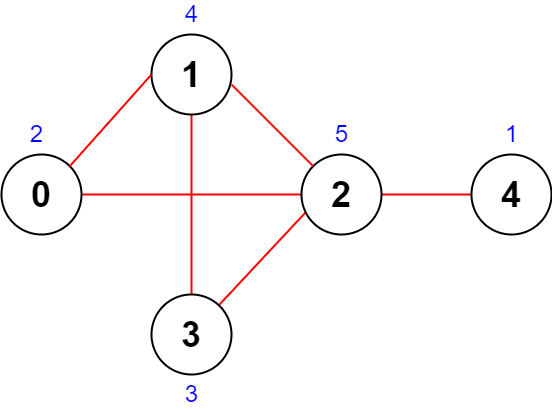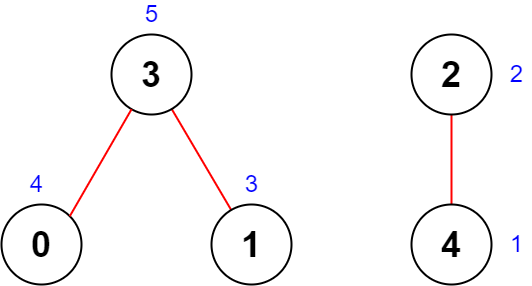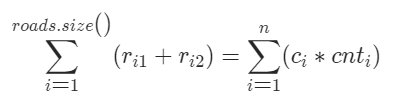## 题目描述

leetcode2285.道路的最大总重要性```输入：n = 5, roads = [[0,1],[1,2],[2,3],[0,2],[1,3],[2,4]]

- 道路 (0,1) 重要性为 2 + 4 = 6 。
- 道路 (1,2) 重要性为 4 + 5 = 9 。
- 道路 (2,3) 重要性为 5 + 3 = 8 。
- 道路 (0,2) 重要性为 2 + 5 = 7 。
- 道路 (1,3) 重要性为 4 + 3 = 7 。
- 道路 (2,4) 重要性为 5 + 1 = 6 。

``````输入：n = 5, roads = [[0,3],[2,4],[1,3]]

- 道路 (0,3) 重要性为 4 + 5 = 9 。
- 道路 (2,4) 重要性为 2 + 1 = 3 。
- 道路 (1,3) 重要性为 3 + 5 = 8 。

```

• `2 <= n <= 5 * 104`
• `1 <= roads.length <= 5 * 104`
• `roads[i].length == 2`
• `0 <= ai, bi <= n - 1`
• `ai != bi`
• 没有重复道路。

## 题解——哈希表

### 思路

`ri`表示道路,`ci`表示城市,`cnt`表示城市连接的道路数,则道路重要性之和可以这样表示